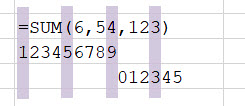# xlf QandA series

## Change argument value

### QUESTION

I NEED A VBA PROCEDURE THAT CAN HELP ME TO CHANGE ONE OF THE ARGUMENTS IN AN EXISTING WS FUNCTION

For example I have arguments like =XXX(3,3,3), and need to change the last "3" into "1". Manually changing every function argument is an option but unfortunately I have 100s of them. [Sean, 29 July 2020]

###Answer

Let =XXX(3,3,3), from the question example, be =SUM(6,54,123) in the code 1 demonstration. The formula character count is shown in figure 1, with 14 characters in total.Fig 1: Example formula - character count by position

## VBA :: xlfReplaceSpecificArgument

An argument is the value passed to the function. The code 1 macro replaces the value of a specific argument in a WS function. The example changes =SUM(6,54,123) to =SUM(6,54,321).

Code 1: Macro xlfReplaceSpecificArgument using InStr (left to right) - returns the position from the start of the string, or specified position
Sub xlfReplaceSpecificArgument()
Dim Item As Range
Dim strFormula As String
Dim intComma1 As Integer, intComma2 As Integer
Dim i As Long

For Each Item In Sheets("Demo").UsedRange
If Item.HasFormula Then
strFormula = Item.Formula
If Left(strFormula, 5) = "=SUM(" Then
intComma1 = VBA.InStr(1, String1:=strFormula, String2:=",")
intComma2 = VBA.InStr(intComma1 + 1, String1:=strFormula, String2:=",")
Item.Formula = Left(strFormula, intComma2) & "321)" ' replace 3rd argument
i = i + 1
End If
End If
Next Item

Debug.Print i, "Formulas replaced"

End Sub


About Code 1

1. Line 12: HasFormula property returns Variant TRUE if cell has formula
2. Line 13: Formula property returns the formula as a String in A1-style notation, "=SUM(6,54,123)". The value is assigned to strFormula variable
3. Line 15: Using the inStr function start at character 1, and search strFormula for the first occurrence of a comma ",". Returns 7, assign to intComma1.
4. Line 16: Using the inStr function start at character 8 (7 + 1), and search strFormula for the first occurrence of a comma ",". Returns 10, assign to intComma2.
5. Line 17:   Concatenate the 10 left characters from strFormula =SUM(6,54, and 321) to form =SUM(6,54,321), then assign formula property to Item object

Alternate code: Macro xlfReplaceSpecificArgument using InStrRev (right to left) - returns the position from the end of the string
'...
If Left(strFormula, 5) = "=SUM(" Then
intComma = VBA.InStrRev(strFormula, ",")
' inStrRev (line 15) -- [Rev]erse (right to left)
Item.Formula = Left(strFormula, intComma) & "321)" ' replace 3rd argument - after last comma
i = i + 1
'...



1. Line 16: alternate Using the inStrRev function start at the end and search strFormula for the first occurrence of a comma ",". Returns 10, assign to intComma.

• This example was developed in Excel 365 :: VBA 7.1
• Published: 29 July 2020
• Revised: Friday 31st of July 2020 - 09:09 AM, [Australian Eastern Standard Time (EST)]